1592492640

# LightGBM: A Highly-Efficient Gradient Boosting Decision Tree - KDnuggets

LightGBM is a histogram-based algorithm which places continuous values into discrete bins, which leads to faster training and more efficient memory usage. In this piece, we’ll explore LightGBM in depth.
The power of the LightGBM algorithm cannot be taken lightly (pun intended). LightGBM is a distributed and efficient gradient boosting framework that uses tree-based learning. It’s histogram-based and places continuous values into discrete bins, which leads to faster training and more efficient memory usage. In this piece, we’ll explore LightGBM in depth.

#decision trees #gradient boosting #machine learning #python

## Buddha Community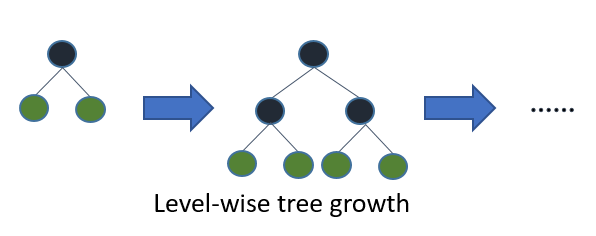1592492640

## LightGBM: A Highly-Efficient Gradient Boosting Decision Tree - KDnuggets

LightGBM is a histogram-based algorithm which places continuous values into discrete bins, which leads to faster training and more efficient memory usage. In this piece, we’ll explore LightGBM in depth.
The power of the LightGBM algorithm cannot be taken lightly (pun intended). LightGBM is a distributed and efficient gradient boosting framework that uses tree-based learning. It’s histogram-based and places continuous values into discrete bins, which leads to faster training and more efficient memory usage. In this piece, we’ll explore LightGBM in depth.

#decision trees #gradient boosting #machine learning #python1596385380

## LightGBM: A Highly-Efficient Gradient Boosting Decision Tree

The power of the LightGBM algorithm cannot be taken lightly (pun intended). LightGBM is a distributed and efficient gradient boosting framework that uses tree-based learning. It’s histogram-based and places continuous values into discrete bins, which leads to faster training and more efficient memory usage. In this piece, we’ll explore LightGBM in depth.

According to the official docs, here are the advantages of the LightGBM framework:

• Faster training speed and higher efficiency
• Lower memory usage
• Better accuracy
• Support of parallel and GPU learning
• Capable of handling large-scale data

## Parameter Tuning

The framework uses a leaf-wise tree growth algorithm, which is unlike many other tree-based algorithms that use depth-wise growth. Leaf-wise tree growth algorithms tend to converge faster than depth-wise ones. However, they tend to be more prone to overfitting.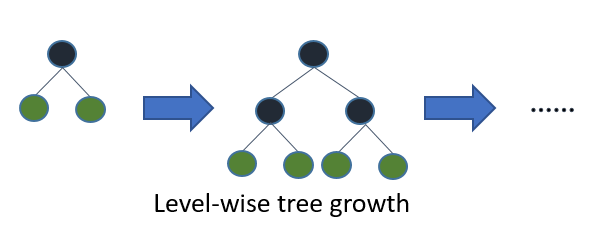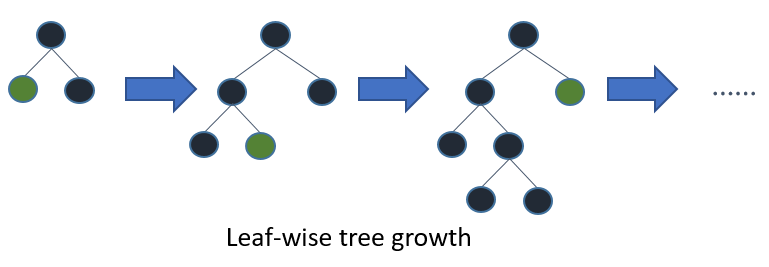Here are the parameters we need to tune to get good results on a leaf-wise tree algorithm:

• `num_leaves`: Setting the number of leaves to `num_leaves = 2^(max_depth)` will give you the same number of leaves as a depth-wise tree. However, it isn’t a good practice. Ideally, the number of leaves should be smaller than `2^(max_depth)`
• `min_data_in_leaf` prevents overfitting. It’s set depending on `num_leaves` and the number of training samples. For a large dataset, it can be set to hundreds or thousands.
• `max_depth` for limiting the depth of the tree.

Faster speeds on the algorithm can be obtained by using:

• a small `max_bin`
• `save_binary` to speed up data loading in future learning
• parallel learning
• bagging, through setting `bagging_freq` and `bagging_fraction`
• `feature_fraction` for feature sub-sampling

In order to get better accuracy, one can use a large `max_bin`, use a small learning rate with large `num_iterations`, and use more training data. One can also use many `num_leaves`, but it may lead to overfitting. Speaking of overfitting, you can deal with it by:

• Increasing `path_smooth`
• Using a larger training set
• Trying `lambda_l1``lambda_l2`, and `min_gain_to_split` for regularization
• Avoid growing a very deep tree

#data-science-for-ml #heartbeat #programming #machine-learning #decision-tree #deep learning1596286260

## Decision Tree -Classification

Decision Tree is one of the most widely used machine learning algorithm. It is a supervised learning algorithm that can perform both classification and regression operations.

As the name suggest, it uses a tree like structure to make decisions on the given dataset. Each internal node of the tree represent a “decision” taken by the model based on any of our attributes. From this decision, we can seperate classes or predict values.

Let’s look at both classification and regression operations one by one.

# Classification

In Classification, each leaf node of our decision tree represents a **class **based on the decisions we make on attributes at internal nodes.

To understand it more properly let us look at an example. I have used the Iris Flower Dataset from sklearn library. You can refer the complete code on Github — Here.

Image for post

A node’s samples attribute counts how many training instances it applies to. For example, 100 training instances have a petal width ≤ 2.45 cm .

A node’s value attribute tells you how many training instances of each class this node applies to. For example, the bottom-right node applies to 0 Iris-Setosa, 0 Iris- Versicolor, and 43 Iris-Virginica.

And a node’s gini attribute measures its impurity: a node is “pure” (gini=0) if all training instances it applies to belong to the same class. For example, since the depth-1 left node applies only to Iris-Setosa training instances, it is pure and its gini score is 0.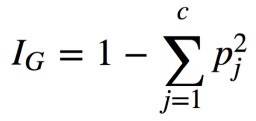Gini Impurity Formula

where, pⱼ is the ratio of instances of class j among all training instances at that node.

Based on the decisions made at each internal node, we can sketch decision boundaries to visualize the model.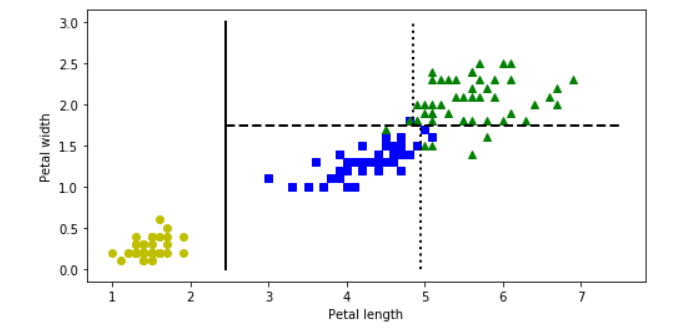But how do we find these boundaries ?

We use Classification And Regression Tree (CART) to find these boundaries.

CART is a simple algorithm that finds an attribute _k _and a threshold _t_ₖat which we get a purest subset. Purest subset means that either of the subsets contain maximum proportion of one particular class. For example, left node at depth-2 has maximum proportion of Iris-Versicolor class i.e 49 of 54. In the _CART cost function, _we split the training set in such a way that we get minimum gini impurity.The CART cost function is given as: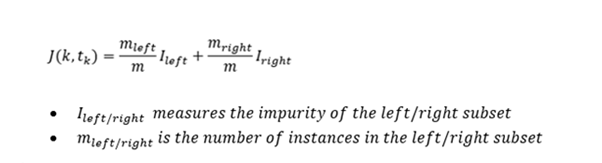After successfully splitting the dataset into two, we repeat the process on either sides of the tree.

We can directly implement Decision tree with the help of Scikit learn library. It has a class called DecisionTreeClassifier which trains the model for us directly and we can adjust the hyperparameters as per our requirements.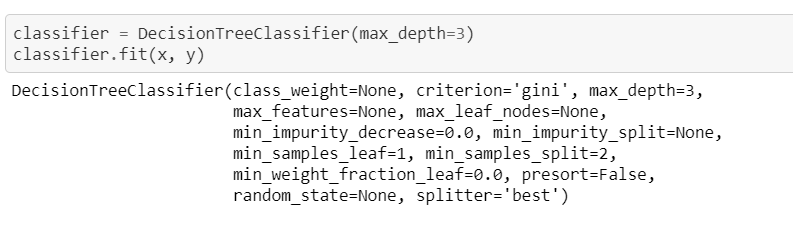#machine-learning #decision-tree #decision-tree-classifier #decision-tree-regressor #deep learning1602882000

## Introduction

Machine learning algorithms require more than just fitting models and making predictions to improve accuracy. Nowadays, most winning models in the industry or in competitions have been using Ensemble Techniques to perform better. One such technique is Gradient Boosting.

This article will mainly focus on understanding how Gradient Boosting Trees works for classification problems. We will also discuss about some important parameters, advantages and disadvantages associated with this method. Before that, let’s get a brief overview of Ensemble methods.

### What are Ensemble Techniques ?

**Bias and Variance **— While building any model, our objective is to optimize both variance and bias but in the real world scenario, one comes at the cost of the other. It is important to understand the trade-off and figure out what suits our use case.

Ensemblesare built on the idea that a collection of weak predictors, when combined together, give a final prediction which performs much better than the individual ones. Ensembles can be of two types —

i) Bagging **— **Bootstrap Aggregation or Bagging is a ML algorithm in which a number of independent predictors are built by taking samples with replacement. The individual outcomes are then combined by average (Regression) or majority voting (Classification) to derive the final prediction. A widely used algorithm in this space is Random Forest.

**ii) Boosting — **Boosting is a ML algorithm in which the weak learners are converted into strong learners. Weak learners are classifiers which always perform slightly better than chance irrespective of the distribution over the training data. In Boosting, the predictions are sequential wherein each subsequent predictor learns from the errors of the previous predictors. Gradient Boosting Trees (GBT) is a commonly used method in this category.1596285180

## Decision Trees Classifier

Both of `Regression Trees` and `Classification Trees` are a part of `CART (Classification And Regression Tree) Algorithm`. As we mentioned in Regression Trees article, tree is composed of 3-major parts; root-node, decision-node and terminal/leaf-node.

The criteria used here in node splitting differs from that being used in Regression Trees. As before we will run our example and then learn how the model is being trained.

There are three commonly measures are used in the attribute selection `Gini impurity` measure, is the one used by `CART` classifier. For more information on these, see Wikipedia.

# Data set being used is `iris` data set

``````import numpy as np
import pandas as pd
from sklearn.tree import DecisionTreeClassifier
from sklearn.tree import export_graphviz
from six import StringIO
from IPython.display import Image
# pip/conda install pydotplus
import pydotplus
from sklearn import datasets
xList = iris.data  # Data will be loaded as an array
labels = iris.target
dataset = pd.DataFrame(data=xList,
columns=iris.feature_names)
dataset['target'] = labels
targetNames = iris.target_names
print(targetNames)
print(dataset)
``````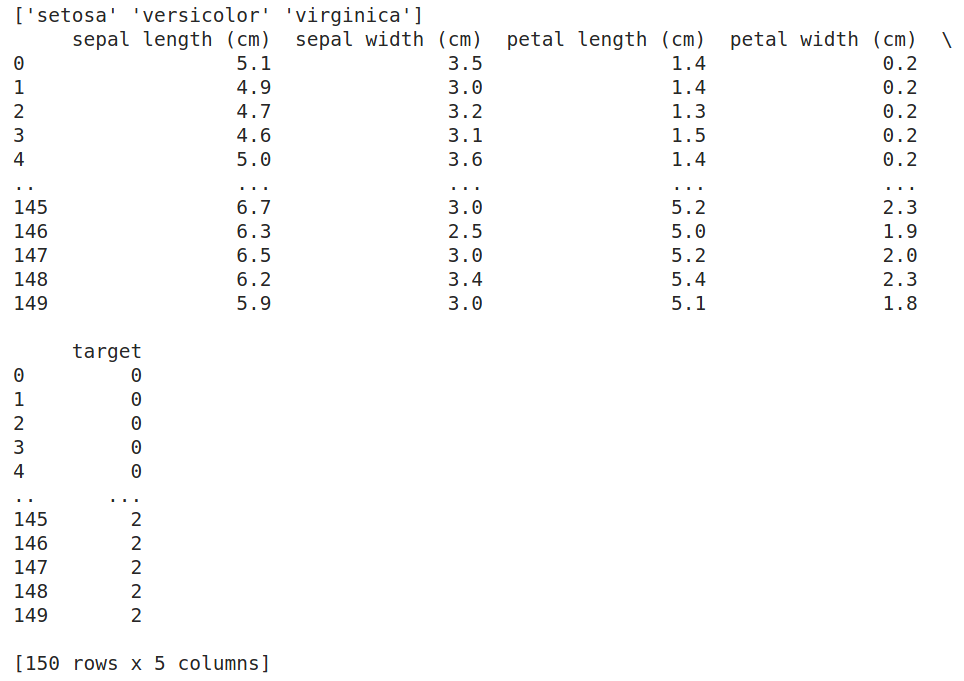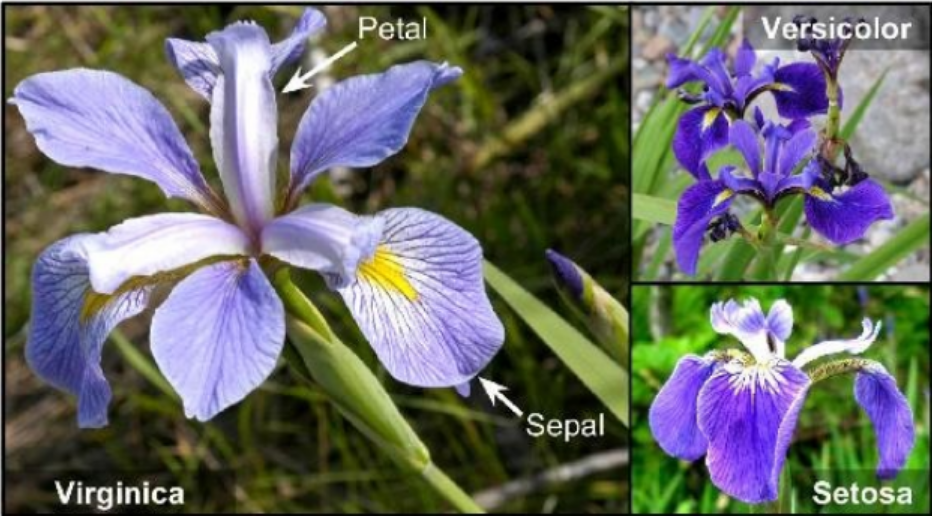Iris Flowers

# How a Binary Decision Tree Generates Predictions

When an observation or row is passed to a non-terminal node, the row answers the node’s question. If it answers yes, the row of attributes is passed to the leaf node below and to the left of the current node. If the row answers no, the row of attributes is passed to the leaf node below and to the right of the current node. The process continues recursively until the row arrives at a terminal (that is, leaf) node where a prediction value is assigned to the row. The value assigned by the leaf node is the mean of the outcomes of the all the training observations that wound up in the leaf node.

# How to split the nodes

Classification trees split a node into two sub-nodes. Splitting into sub-nodes will increase the homogeneity of resultant sub-nodes. In other words, we can say that the purity of the node increases with respect to the target variable. The decision tree splits the nodes on all available variables and then selects the split which results in most homogeneous/pure sub-nodes.

There are major measures being used to determine which attribute/feature is used for splitting and which value within this attribute we will start with. Some of these measures are:

• Gini index (Default in SciKit Learn Classifier).
• Entropy.
• Information gain.

We will start with `Gini index` measure and try to understand it

# Gini Index

Gini index is an impurity measure used to evaluate splits in the dataset. It is calculated by getting the sum of the squared probabilities of each class (target-class) within a certain attribute/feature then benig subtacted from one.

#machine-learning #mls #decision-tree #decision-tree-classifier #classification #deep learning